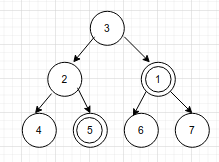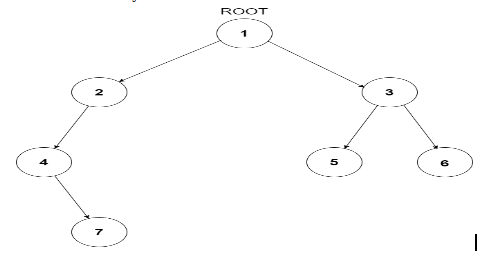2

# Least Common Ancestor

Difficulty: MEDIUMContributed By
Achint Narang
Avg. time to solve
25 min
Success Rate
75%

Problem Statement
Suggest Edit

#### For example look at the tree below, the LCA of node 1 and 5 is 3.#### Note :

``````You have to return the deepest node which has both x, y as its descendants.
There may be cases where one of u or v is not present in the tree. In those cases, the reference provided to u or v will be NULL.
``````
##### Input Format :
``````The first line of the input contains a single integer T, representing the number of test cases.

The first and only line of each test case contains the values of the nodes of the tree in the level order form ( -1 for NULL node) Refer to the example for further clarification.
``````

Example: Consider the binary tree##### The input of the tree shown in the above image will look like:
``````1 2 3 4 -1 5 6 -1 7 -1 -1 -1 -1 -1 -1

Explanation :
Level 1 :
The root node of the tree is 1

Level 2 :
Left child of 1 = 2
Right child of 1 = 3

Level 3 :
Left child of 2 = 4
Right child of 2 = null (-1)
Left child of 3 = 5
Right child of 3 = 6

Level 4 :
Left child of 4 = null (-1)
Right child of 4 = 7
Left child of 5 = null (-1)
Right child of 5 = null (-1)
Left child of 6 = null (-1)
Right child of 6 = null (-1)

Level 5 :
Left child of 7 = null (-1)
Right child of 7 = null (-1)

The first not-null node (of the previous level) is treated as the parent of the first two nodes of the current level. The second not-null node (of the previous level) is treated as the parent node for the next two nodes of the current level and so on. The input ends when all nodes at the last level are null (-1).
``````
##### Output Format :
``````For each test case, print the LCA of the given nodes in a separate line.
``````
##### Note :
``````You do not need to print anything. It has already been taken care of. Just implement the given function.
``````
##### Constraints :
``````1 <= T <= 50
1 <= N <= 10^4
1 <= nodeVal <= 10^9

Time Limit: 1 sec
``````
##### Sample Input 1 :
``````2
1 2 3 4 -1 5 6 -1 7 -1 -1 -1 -1 -1 -1
7 5
1 2 3 4 -1 5 6 -1 7 -1 -1 -1 -1 -1 -1
4 2
``````
##### Sample Output 1 :
``````1
2
``````
##### Explanation for Sample Output 1 :
``````For the first test case, as shown in the above figure, the root of the tree is the deepest node which contains both the nodes 7 and 5 as its descendants, hence 1 is the answer.
For the second test case, since 4 is one of the children of 2, so 2 is the answer.
``````
##### Sample Input 2 :
``````2
1 2 -1 3 -1 -1 -1
3 1
9 -1 -1
9 9
``````
##### Sample Output 2 :
``````1
9
``````Console